Equations of Lines

1. The slope of a line through the points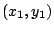andis given by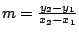.

2. Point-Slope Form

The line through the pointwith slope m has the equation.

3. Slope-Intercept Form

The line with slope m and y-intercept b has equation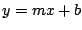.

4. Two lines with slopesandare

parallel iffperpendicular iff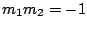.

Ex 1 Find an equation of the line which passes through the pointand has slope 3.

Sol Using the Point-Slope Form, we obtain the equationor, simplifying,or.

If instead we use the Slope-Intercept Form, we get the equation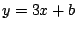. To determine b, we substituteand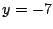to obtain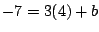, so that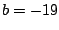.

Ex 2 Find an equation of the line which passes through the point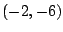and is parallel to the line.

Sol To find the slope of the line, solve for y to get the equation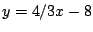; so this line has slope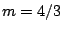. Since the line we are seeking is parallel to this line, its slope is also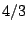, so its equation is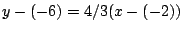or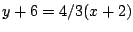or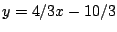.

Pr 1 Find an equation of the line which passes through the pointand has y-intercept 5.

Pr 2 Find an equation of the line which passes through the pointsand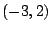.

Pr 3 Find an equation of the line which passes through the pointsand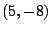.

Pr 4 Find an equation of the line which passes through the point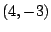and is perpendicular to the line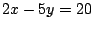.

Pr 5 Find an equation of the perpendicular bisector of the line segment between the points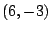and.

Pr 6 Find the slope-intercept form of the equation of the line which passes through the pointand which is parallel to the line through the pointsand.

Pr 7 Find an equation of the tangent line to the circleat the point.

Pr 8 Find the point of intersection of the lines with equationsand.